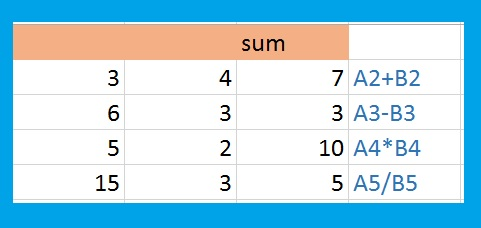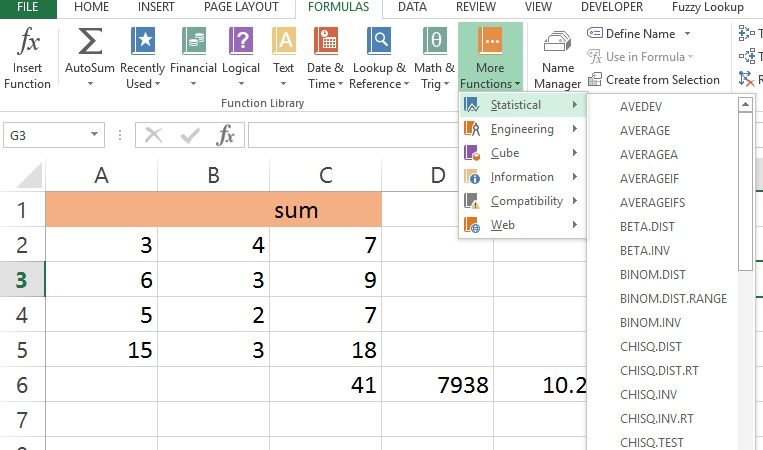# Excel: Learning to Use Formulas

The first step towards being an Excel Power User is learning to use formulas. Pulling out a calculator to add up Excel columns is a sure fire way to let everyone in the office know your computer skills are lacking. Luckily for you, basic Excel formulas are pretty easy learn.

## Lesson 1: Basic Arithmetic Formulas

Video Link: Excel Formulas Part 1

Excel supports all standard Arithmetic functions – addition, subtraction, multiplication, division, etc. **Excel comes standard with advanced engineering and statistical functions, but that is well beyond the scope of this article.

To start a formula in Excel: select the cell where the result belongs and type =. Using = as the first character tells Excel you want to create a formula.

In this example, we want to add the first row of column A and B and place the answer in C. Select cell C2, enter =A2+B2. Press enter and 7 will appear in the space. Try changing the values in cells A2 and B2. Notice the value in C2 changes in kind.Notice how as you type in a formula, it populates in the formula bar above as well. You can work in the formula bar instead if you chose (as your formulas get longer and more complex, you will find yourself doing more work in the formula bar).

You can try out some other arithmetic functions following the example below: **note: make sure to start your formulas with =## Lesson 2: Replicating Formulas

Video Link: Excel Formulas Part 2

If you had to input each formula individually every time you wanted to use one, this would not be any more efficient then simply doing the calculations on your old desktop calculator. Fortunately, Excel makes replicating your formulas across many cells as simple as clicking a mouse button.

In the bottom right corner of an active cell, there is a small green box. You can click on this box and drag it over the cells you would like the formula to replicate. Or, you can just double click corner and it will automatically replicate the remaining rows in your table.## Lesson 3: Functions

Excel has hundreds of built in functions (far too many to cover in this article). These functions save you having to write complex formulas manually.

Below are examples of 3 commonly used functions (SUM, PRODUCT, AVERAGE)Let us break these functions down:

1. SUM() — adds the values of all cells listed in the parenthesis.  **In the example above, the SUM function has C2:C5 in the parenthesis. The colon(:) means between. So C2:C5 actually means all the values C2 through and including C5 (C2,C3,C4,C5)
2. PRODUCT() — multiplies the values of all cells listed in the parenthesis.
3. AVERAGE() — takes the average of the values of all cells listed in the parenthesis.

If you are curious as to what other built in functions Excel offers, go to the Formulas tab in the ribbon cable.